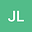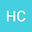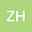Infinitely many solutions for quasilinear schr\”{o}dinger equation with general superlinear nonlinearity
•••• +1
• Jiameng Li,
• Huiwen Chen,
• Zhimin He,
• Zigen Ouyang
Jiameng Li
University of South China

Corresponding Author:1757216006@qq.com

Author ProfileHuiwen Chen
University of South China
Author ProfileZhimin He
Central South University
Author ProfileZigen Ouyang
University of South China
Author Profile## Abstract

In this article, we study the quasilinear Schr\”{o}dinger equation \begin{eqnarray*} \begin{array}{ll} \triangle{u}+V(x)u-\triangle(u^2)u=g(x,u), \ x\in\mathbb{R}^N, \end{array} \end{eqnarray*} where the potential $V(x)$ and the primitive of $g(x,u)$ is allowed to be sign-changing. Under more general superlinear conditions on $g$, we obtain the existence of infinitely many nontrivial solutions by using Mountain Pass Theorem. Recent results in the literature are significantly improved.